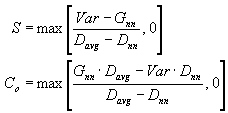# Default Linear Variogram

The default linear variogram takes the form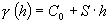where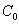is the unknown nugget effect, and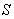is the unknown slope. We need two defining equations to solve for these two unknown parameters.

According to theory, the expected value of the sample variance is the average value of the variogram between all possible pairs of sample locations (Barnes, 1991); this yields one equation. The second equation is generated by equating the experimental sample variogram for nearest neighbors to the modeled variogram. Thus, we can write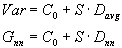where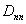= average distance to the nearest neighbor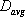= average inter-sample separation distance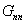= one half the averaged squared difference between nearest neighbors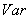= sample variance

By solving the two equations for the two unknown parameters, and checking for unreasonable values, we get the final formulae used in Surfer: